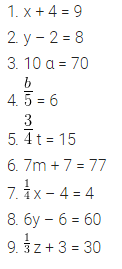# Write equations for the following statements. The sum of numbers x and 4 is 9

Write equations for the following statements.

1. The sum of numbers x and 4 is 9.
2. 2 subtracted from y is 8.
3. Ten times a is 70.
4. The number b divided by 5 gives 6.
5. Three-fourth oft is 15.
6. Seven times m plus 7 gets you 77.
7. One-fourth of a number x minus 4 gives 4.
8. If you take away 6 from 6 times y, you get 60.
9. If you add 3 to one-third of z, you get 30.